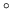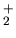## 4.2.1 Angular scattering

The angular scattering was measured using the position sensitive detector (PSD) at the ILENA setup (Figure 2.1). Using integration times of 60 to 180 seconds reasonable statistics were obtained. Normally data gathered to determine the ionization efficiency was used. The raw data was then further processed using the IDL script beamshape to determine the scattering profile. This script directly transforms the data into contour plots. The data was corrected for the blind spot resulting from the (for some regions of the image) very small angle between the particle trajectory and the MCP channel axis as described in . No correction was applied for the energy dependent detection efficiency of the MCP.

The standard angle of incidence of the primary beam was 82. This value was chosen for compatibility with prior measurements done with the ILENA apparatus. In this work only Oprimary particles were used. The molecules would be neutralized prior to reflection by the surface. The scattering properties were measured using the total reflected particle flux and also using the neutral fraction only. Key values calculated from these scattering profiles were the full width half maximum FWHM angles in polar and azimuthal direction and the reflection efficiency (explained below). Most of the measurements were done at 390eV per atom except for the CVD diamond and the MgO (100) sample where the energy dependence of the scattering was investigated.

March 2001 - Martin Wieser, Physikalisches Institut, University of Berne, Switzerland